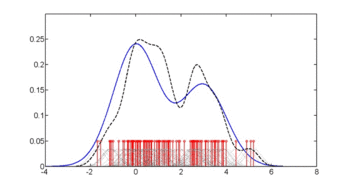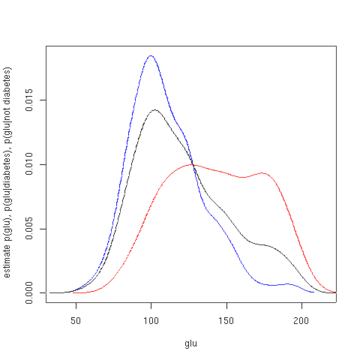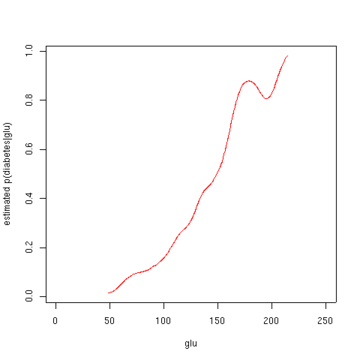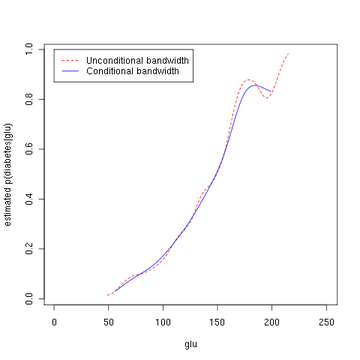# Density estimation

For the signal processing concept, see spectral density estimation.Demonstration of density estimation using kernel smoothing: The true density is mixture of two Gaussians centered around 0 and 3, shown with solid blue curve. In each frame, 100 samples are generated from the distribution, shown in red. Centered on each sample, a Gaussian kernel is drawn in gray. Averaging the Gaussians yields the density estimate shown in the dashed black curve.

In probability and statistics, density estimation is the construction of an estimate, based on observed data, of an unobservable underlying probability density function. The unobservable density function is thought of as the density according to which a large population is distributed; the data are usually thought of as a random sample from that population.

A variety of approaches to density estimation are used, including Parzen windows and a range of data clustering techniques, including vector quantization. The most basic form of density estimation is a rescaled histogram.

## Example of density estimation

We will consider records of the incidence of diabetes. The following is quoted verbatim from the data set description:

A population of women who were at least 21 years old, of Pima Indian heritage and living near Phoenix, Arizona, was tested for diabetes mellitus according to World Health Organization criteria. The data were collected by the US National Institute of Diabetes and Digestive and Kidney Diseases. We used the 532 complete records.

In this example, we construct three density estimates for "glu" (plasma glucose concentration), one conditional on the presence of diabetes, the second conditional on the absence of diabetes, and the third not conditional on diabetes. The conditional density estimates are then used to construct the probability of diabetes conditional on "glu".

The "glu" data were obtained from the MASS package of the R programming language. Within R, ?Pima.tr and ?Pima.te give a fuller account of the data.

The mean of "glu" in the diabetes cases is 143.1 and the standard deviation is 31.26. The mean of "glu" in the non-diabetes cases is 110.0 and the standard deviation is 24.29. From this we see that, in this data set, diabetes cases are associated with greater levels of "glu". This will be made clearer by plots of the estimated density functions.

The first figure shows density estimates of p(glu | diabetes=1), p(glu | diabetes=0), and p(glu). The density estimates are kernel density estimates using a Gaussian kernel. That is, a Gaussian density function is placed at each data point, and the sum of the density functions is computed over the range of the data.diabetes=0) (blue), and p (glu) (black)

From the density of "glu" conditional on diabetes, we can obtain the probability of diabetes conditional on "glu" via Bayes' rule. For brevity, "diabetes" is abbreviated "db." in this formula.The second figure shows the estimated posterior probability p(diabetes=1 | glu). From these data, it appears that an increased level of "glu" is associated with diabetes.glu)

### Script for example

The following R commands will create the figures shown above. These commands can be entered at the command prompt by using cut and paste.

library(MASS)
data(Pima.tr)
data(Pima.te)

Pima <- rbind (Pima.tr, Pima.te)
glu  <- Pima[, 'glu']

d0 <- Pima[, 'type'] == 'No'
d1 <- Pima[, 'type'] == 'Yes'
base.rate.d1 <- sum(d1) / (sum(d1) + sum(d0))

glu.density    <- density (glu)
glu.d0.density <- density (glu[d0])
glu.d1.density <- density (glu[d1])

glu.d0.f <- approxfun(glu.d0.density$x, glu.d0.density$y)
glu.d1.f <- approxfun(glu.d1.density$x, glu.d1.density$y)

p.d.given.glu <- function(glu, base.rate.d1)
{
p1 <- glu.d1.f(glu) * base.rate.d1
p0 <- glu.d0.f(glu) * (1 - base.rate.d1)
p1 / (p0 + p1)
}

x <- 1:250
y <- p.d.given.glu (x, base.rate.d1)
plot(x, y, type='l', col='red', xlab='glu', ylab='estimated p(diabetes|glu)')

plot(density(glu[d0]), col='blue', xlab='glu', ylab='estimate p(glu),
p(glu|diabetes), p(glu|not diabetes)', main=NA)
lines(density(glu[d1]), col='red')


Note that the above conditional density estimator uses bandwidths that are optimal for unconditional densities. Alternatively, one could use the method of Hall, Racine and Li (2004) and the R np package for automatic (data-driven) bandwidth selection that is optimal for conditional density estimates; see the np vignette for an introduction to the np package. The following R commands use the npcdens() function to deliver optimal smoothing. Note that the response "Yes"/"No" is a factor.

library(np)

fy.x <- npcdens(type~glu, nmulti=1, data=Pima)

Pima.eval <- data.frame(type=factor("Yes"),
glu=seq(min(Pima$glu), max(Pima$glu), length=250))

plot(x, y, type='l', lty=2, col='red', xlab='glu',
ylab='estimated p(diabetes|glu)')
lines(Pima.eval\$glu, predict(fy.x, newdata=Pima.eval), col="blue")
legend(0, 1, c("Unconditional bandwidth", "Conditional bandwidth"),
col=c("red", "blue"), lty=c(2, 1))


The third figure uses optimal smoothing via the method of Hall, Racine, and Li indicating that the unconditional density bandwidth used in the second figure above yields a conditional density estimate that may be somewhat undersmoothed.glu)

1. Smith, J. W., Everhart, J. E., Dickson, W. C., Knowler, W. C. and Johannes, R. S. (1988). R. A. Greenes, ed. "Using the ADAP learning algorithm to forecast the onset of diabetes mellitus". Proceedings of the Symposium on Computer Applications in Medical Care (Washington, 1988). Los Alamitos, CA: IEEE Computer Society Press: 261–265. PMC 2245318.